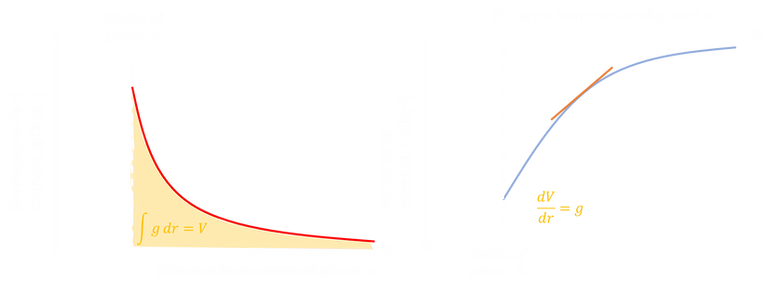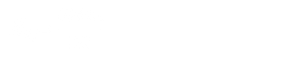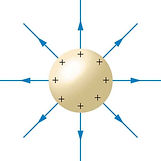top of page# 10.2. Fields at Work

It takes a phenomenal amount of energy to put a rocket into space. There is a nice gif that did the rounds a while back that visualises the launch of the Saturn V rocket using the equivalent mass of elephants. Back when the space shuttle was in operation, it cost about \$55 000 for each kilogram put into orbit - a large fraction of which would be due to the fuel. Now with SpaceX we have got this down to about \$3 000 per kg.  Clearly we need to do a lot of work in order to increase the gravitational potential energy of the rocket, and this comes from the rocket fuel. The engineering behind rocket design is fascinating - and this video illustrates just one small component, the noise suppression system. With such a huge volume of fuel being ignited at once, the sound generated from this would be sufficient to cause significant infrastructure damage (not to mention killing any onlookers), so the NASA engineers designed a noise suppression system to prevent this.

This section looks at the work and energy associated with movement in fields - whether that be masses in gravitational fields or charges in electric fields, the mathematics is very similar. It has been divided up as follows:

Potential & Potential Energy

#### Potential and Potential Energy

In Section 10.1 we looked at a few key ideas related to electric and gravitational fields and forces. We established the fact that both gravitational and electric fields get weaker (with an inverse square relationship) as we move further away. Now we will move on to look at work done and energy transfers as we move within a field.

The video below is a nice little introduction to thinking about these key ideas in relation to energy transfers - how much energy does it take to get into space?

## Potential Energy and Work Done

Let's now think about the work done in launching a rocket from the surface of the Earth. How does the work done PER METRE required to move this rocket depend on how far away we are? We are doing work AGAINST the gravitational field to launch our rocket, so if the gravitational field gets weaker the further away we are, the lower the work done required to move the same distance.

How can we work this out quantitatively? Let’s start with our inverse square Force-Distance graph we have looked at before. As Work done = Force x Distance moved, finding the area under this curve will tell us the ttal energy transferred (notice we did something similar with our Hooke’s law stuff way back in Section 2.3).We can mathematically find the area by integrating our gravitational force equation with respect to distance.

We are looking at the work done required to move our rocket from the planet’s surface to outside the influence of the Earth’s field (which as we know is infinite), so let’s set our integration limits as R and ∞.## Potential Energy and Infinity as a reference point

In physics, sign (+ or -) normally indicates a direction, and therefore is a property of a vector. Energy is a scalar and therefore, strictly, whilst it can be zero, it cannot be negative… there is either “some” energy or there is none.

However, in the vast majority of situations we are only ever concerned about changes in potential energy rather than the total amount (which is very difficult and relatively pointless to calculate in most cases anyway).

Therefore, often a reference point will be chosen for zero, which is not actually the zero point. Therefore it can appear that an object has a negative potential energy, but this actually just means less than the reference point. (We already saw this previously when talking about energy levels in atoms).

The only point in the universe that really makes sense, therefore, for our reference point is where an object has maximum GPE, as far away from every star, planet, galaxy as possible - infinity

We therefore define our potential energy as follows:

The work done in moving an object from infinity to that point in space.

As work is done BY the field in moving towards the planet, this always gives a negative number.This means that our equation for gravitational potential energy is given below (notice the addition of the minus sign). Importantly, this also follows in inverse relationship, where E ∝ 1/r (rather than inverse square as with force).## Electric Potential Energy and Potential

There are plenty of similarities between gravitational and electric fields, so let's now look at how the electrical potential energy changes as we moved a charged particle within an electric field. The key differece to remember is that the force can be attractive or repulsive.

Going back to our definition of potential energy:

The work done (against the field) in moving an object from infinity to that point in space.

(Remember our reference for electrostatic fields is a point positive test charge).

Two key points:

• If we bring a negative charge from infinity to the surface of our reference charge, work is done BY the field, therefore the potential energy is negative (i.e. attractive forces, similar to the shape of our GPE graph).

• If we bring a positive charge from infinity to the surface of our reference charge, work is done AGAINST the field, therefore the potential energy is positive (i.e. repulsive forces).## Video Lessons

 Gradepod Gravititional Potential IB Specific Khan Academy Electric Potential Energy Science Shorts Gravitational and Electric Fields (Potential)

## Resources

 IB Physics Topic 10 Notes IB-Physics.net Chapter 10 Summary IB Revision Notes Isaac Physics Gravitational Potential Level 5 beyond IB Mr. G 10.2 Teaching Notes 10.2 Student Notes Physics and Maths Tutor Fields Definitions Fields Key Points Fields Detailed Notes Fields Flashcards A Level Resources - content slightly different

## Questions

 Cambridge University Press Topic 10: MCQs CUP Website Link Freely available online Grade Gorilla 10 (Fields) MCQs Topic 10 (Fields) Final Quiz Quick IB Specific Mixed MCQs Isaac Physics Energies and Potentials of Charges Mr. G 10.2 Formative Assessment Topic 10 Summary Qs IB Specific Questions

#### Potential Gradient and Potential Difference

We talked about electric potential in the previous section. If we look at the field between two plates, we see the equipotential lines between two charged plates. Each equipotential line represents points of a constant potential.Now that we have looked mathematically in a few conventions related to potential energy, let's now look more closely at this term potential.

We found our potential energy by integrating our gravitational force with respect to distance.We can follow this same integration process with our gravitational field strength to obtain a new term, called gravitational potential, V, with units of Joules per kilogram

This is defined as :

The work done per unit mass in moving a point mass from infinity to that point in space.

Notice that this is very similar to our equation for EP, but divided by our mass. This makes potential a really useful property when studying fields, as we can calculate how much energy is needed PER UNIT MASS to move from one point in a field to another.

Note the following relationships:Graphically, this follows the same inverse relationship as GPE, with V ∝ - 1/r.

We calculated our gravitational potential by integrating our gravitational field strength with reps;respect to distance.

Reversing this process we can differentiate our gravitational potential to find our field strength. Looking at our potential graph on the right below, we can see that the field strength is greatest where the graph is steepest. Therefore, our gravitational field strength at a certain point is also known as the POTENTIAL GRADIENT.## Electric Potential

As before, we can look at our equivalent in moving charges within an electric field. Rather than using a unit mass, we will instead look at a test charge of +1 C.

We therefore define our electric potential as :

The work done per unit charge in moving a point positive test charge from infinity to that point in space.

Our therefore equation looks like this.Graphically we have the same positive and negative relationship we did before.Going back to this simulation by Geogebra and we can se much more clearly this concept of potential gradient. The steeper the gradient of the potential, the stronger the electric field at that point, and the closer together the equipotential lines.

## Video Lessons

 Khan Academy Electric Potential at a point

## Resources

 IB Physics Topic 10 Notes IB-Physics.net Chapter 10 Summary IB Revision Notes Mr. G 10.2 Teaching Notes 10.2 Student Notes Physics and Maths Tutor Fields Definitions Fields Key Points Fields Detailed Notes Fields Flashcards A Level Resources - content slightly different

## Questions

 Cambridge University Press Topic 10: MCQs CUP Website Link Freely available online Grade Gorilla 10 (Fields) MCQs Topic 10 (Fields) Final Quiz Quick IB Specific Mixed MCQs Mr. G 10.2 Formative Assessment Topic 10 Summary Qs IB Specific Questions
Escape Velocity

#### Escape Velocity

How fast do you need to move to escape from the gravitational pull of Earth? Well, if something is given an initial burst of kinetic energy, as it moves further from the surface of Earth it will slow down, and kinetic energy will be transferred into gravitational potential energy.

We can use this idea to work out the kinetic energy needed to get to get to infinity, by equating our EK and EP.

We can use this idea to work out the kinetic energy needed to get to get to infinity, by equating our EK and EP.If we substitute in values for Earth, with M = 6 x 10²⁴ kg and r = 6400 km, we can work out the escape velocity required to leave the Earth's gravitational pull - which works out at about 11 kmsˉ¹. What this means is that something projected with this initial velocity should have enough kinetic energy to reach an infinite distance from the surface of the Earth.

## Video Lessons

 Khan Academy Gravity at Large Distances

## Resources

 IB Physics Topic 10 Notes IB-Physics.net Chapter 10 Summary IB Revision Notes Mr. G 10.2 Teaching Notes 10.2 Student Notes Physics and Maths Tutor Fields Definitions Fields Key Points Fields Detailed Notes Fields Flashcards A Level Resources - content slightly different

## Questions

 Cambridge University Press Topic 10: MCQs CUP Website Link Freely available online Grade Gorilla 10 (Fields) MCQs Topic 10 (Fields) Final Quiz Quick IB Specific Mixed MCQs Mr. G 10.2 Formative Assessment Topic 10 Summary Qs IB Specific Questions
Orbital Motion

#### Orbital Motion

We can combine our ideas about circular motion and potential to understand a few ideas about satellites in orbit around a massive body. Scishow have a nice little video to introduce this subsection:

Let's look at the energy of a satellite in orbit. It will have both gravitational and potential energy.   We've already looked at the gravitational potential energy, as below:In Section 6.2. we looked at objects in orbit. We worked out our orbital velocity by equating our Newton's Law of Gravitation and centripetal force equations.By subsituting into our equation for kinetic energy, we obtain the following:Lastly, we can work out the total energy of the satellite in orbit by summing our potential and kinetic energies.Showing these three equations on a graph we obtain the following.This graph can tell us some useful ideas about what happens when we put a satellite into a different orbit.

If we put satellite into a higher orbit:

• It's total energy increases, so we need to do work to increase the orbital radius

• It's potential energy increases, as it is further from the surface of the planet

• It's kinetic energy DECREASES, meaning it travels slower at this new orbit

Inside the Planet

## Inside a massive sphere

We've talked about how gravitational potential and field strength changes as we move from infinity to the surface of a planet. But what happens then as we move from the surface to the interior of the planet? At the surface of Earth we have a gravitational field strength of 9.81 Nkgˉ¹, pulling you downwards towards the centre of the planet. If we were to teleport to the centre of the Earth, the mass of the planet would be spread evenly around us, therefore the resultant field strength would be equal to zero.

A classic Physics thought experiments I like to give to my classes is to ask what about happen if you jumped into a hole drilled through the Earth. Minute Physics have a great little video that talks through this.

Our graph of gravitational field strength against distance from the centre is shown below. We see that as we move from the surface of the planet to the centre, the field strength decreases linearly, before reaching zero at the centre.  (this assumes a body of uniform density, which is of course not truly the case with Earth!)## Inside a charged sphere

Now, how do things change if we are talking about a charged conducing sphere?

There is a distinct difference between massive and charged spheres, in that the charges within a conductor are able to redistribute themselves. In a charged conductive sphere, the charges will distribute themselves so they are uniformly dispersed around the surface of the sphere, effectively forming a charged shell as shown on the right.

Within this charged shell, the electric field cancels, resulting in a resultant field strength of zero (below left). As the electric field strength is equal to the potential gradient, this means that the electric potential inside this shell remains constant.